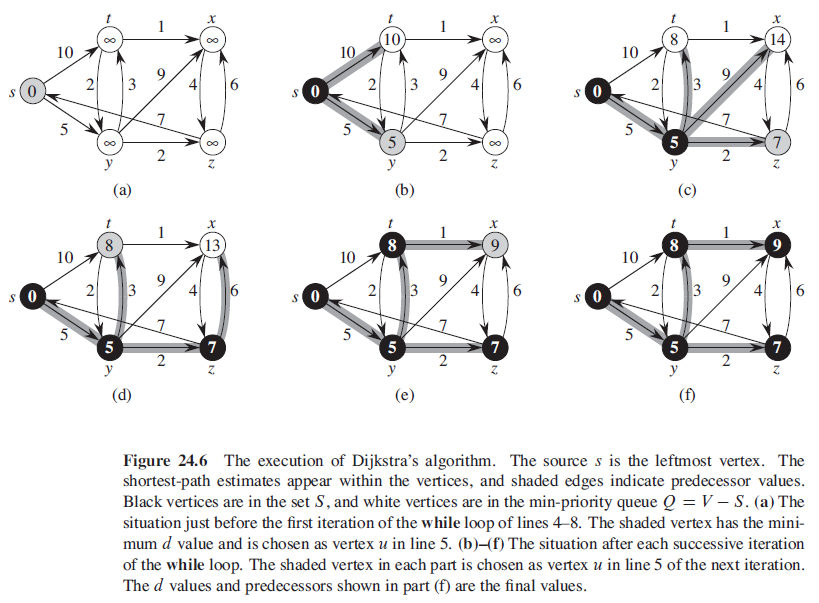## 什么是图

### 图的表示

• 邻接链表：稀疏图
• 邻接矩阵：稠密图，或者需要快速判断任意两个结点之间是否有边的情况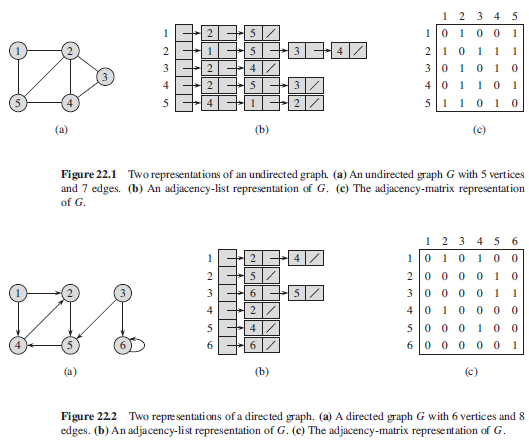## 广度优先搜索

  1 2 3 4 5 6 7 8 9 10 11 12 13 14 15 16 17 18 19 20 21 22 23 24 25 26  /** u.color 存放结点 u 的颜色属性 * 白色表示未被发现 * 灰色表示邻接结点中还有白色结点 * 黑色表示邻接结点均被发现 * u.π 存放前驱结点 * u.d 存放从源结点 s 到结点 u 之间的距离 **/ BFS(G, s) for each vertex u in G.V - {s} u.color = WHITE u.d = ∞ u.π = NIL s.color = BLACK s.d = 0 s.π = NIL Q = ∅ ENQUEUE(Q, s) while Q ≠ ∅ // 灰色结点的集合 u = DEQUEUE(Q) for each v in G.Adj[u] if v.color == WHITE v.color = GRAY v.d = u.d + 1 v.π = u ENQUEUE(Q, v) u.color = BLACK 

BFS 的总运行时间为 O(V + E)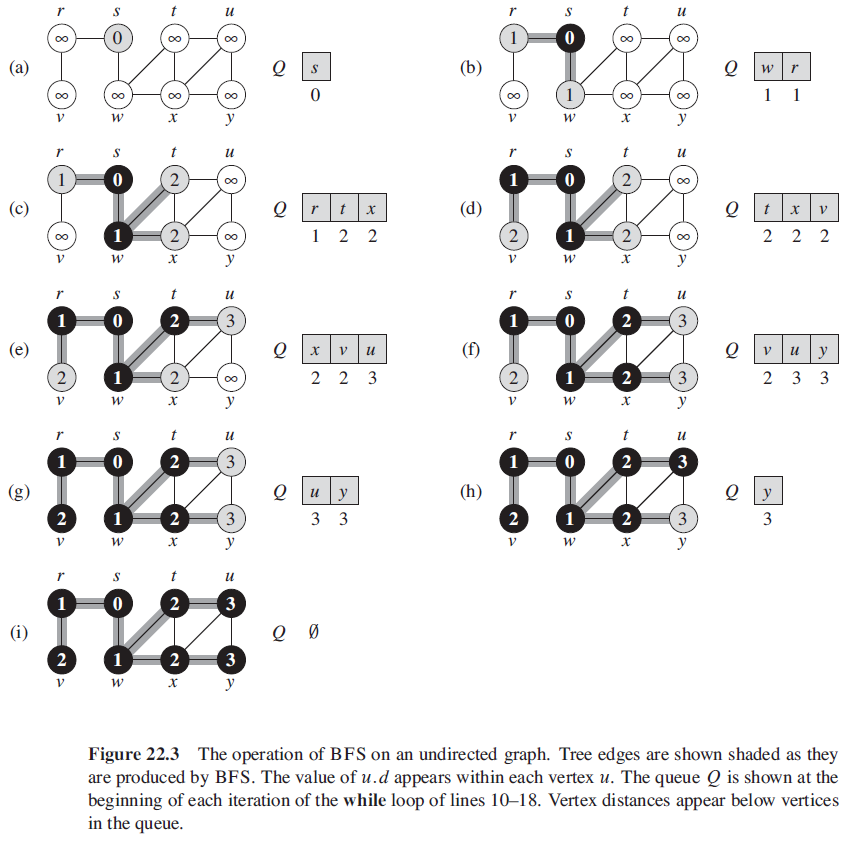### BFS 的性质

1. BFS 可以计算出从源结点 s 到 结点 v 的最短路径。
2. BFS 在对图进行搜索的过程中将创建一棵广度优先树

## 深度优先搜索

  1 2 3 4 5 6 7 8 9 10 11 12 13 14 15 16 17 18 19 20 21 22 23 24 25 26 27 28  /** u.color 存放结点 u 的颜色属性 * 白色表示未被发现 * 灰色表示邻接结点中还有白色结点 * 黑色表示邻接结点均被发现 * u.π 存放前驱结点 * u.d 记录结点 u 第一次被发现的时间 * u.f 记录搜索完成对 u 的邻接链表扫描的事件 **/ DFS(G) for each vertex u in G.V u.color = WHITE u.π = NIL time = 0 for each vertex u in G.V if u.color == WHITE DFS-VISIT(G, u) DFS-VISIT(G, u) time = time + 1 // white vertex u has just been discovered u.d = time u.color = GRAY for each v in G.Adj[u] // explore edge (u, v) if v.color == WHITE v.π = u DFS-VISIT(G, v) u.color = BLACK // blacken u, it is finished time = time + 1 u.f = time 

DFS 的总运行时间为 O(V + E)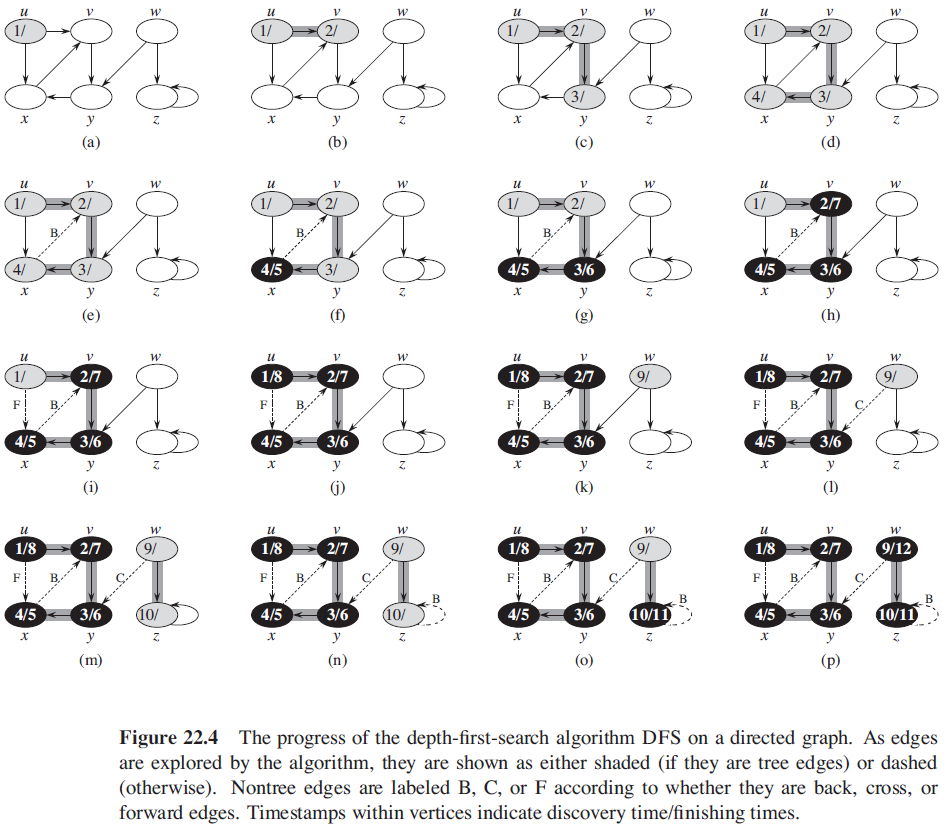### DFS 的性质

1. 括号化定理：在对有向或无向图进行的任意 DFS 中，对于任意两个结点 u 和 v 来说，下面三种情况只有一种成立：

• 区间 $[u.d, u.f]$ 和 区间 $[v.d, v.f$ 完全分离，在深度优先森林中，结点 u 不是结点 v 的后代，结点 v 也不是结点 u 的后代
• 区间 $[u.d, u.f]$ 完全包含在 区间 $[v.d, v.f$ 内，在深度优先树中，结点 u 是结点 v 的后代
• 区间 $[v.d, v.f]$ 完全包含在 区间 $[u.d, u.f$ 内，在深度优先树中，结点 v 是结点 u 的后代
2. 边的分类

• 树边：是深度优先森林 $G_π$ 中的边。如果结点 v 是因算法对边 (u, v) 的探索而首先被发现，则 (u, v) 是一条树边
• 后向边：是将结点 u 连接到其在深度优先树中祖先结点 v 的边。由于有向图中可以有自循环，自循环也被认为是后向边
• 前向边：是将结点 u 连接到其在深度优先树中后代结点 v 的边
• 横向边：其他所有的边

### 拓扑排序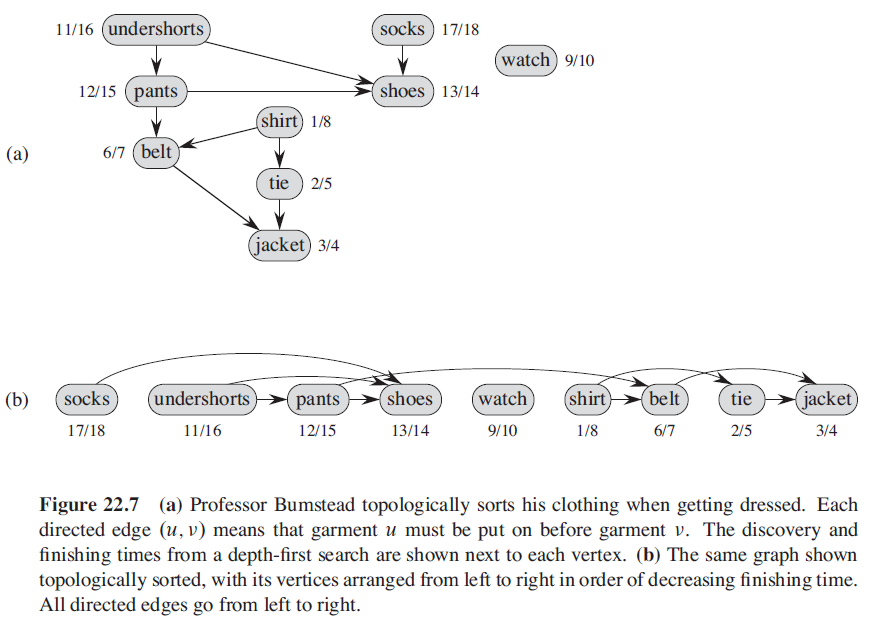1 2 3 4  TOPOLOGICAL-SORT(G) call DFS(G) to compute finishing time v.f for each vertex v as each vertex is finished, insert it onto the front of a linked list return the linked of list of vertices 

### 强连通分量

 1 2 3 4 5 6 7  STRONGLY-CONNECTED-COMPONENTS(G) call DFS(G) to compute finishing time u.f for each vertex u compute G^T call DFS(G^T), but in the main loop of DFS, consider the vertices in order of decreasing u.f (as computed in line 1) output the vertices of each tree in the depth-first forest formed in line 3 as a separate strongly connected component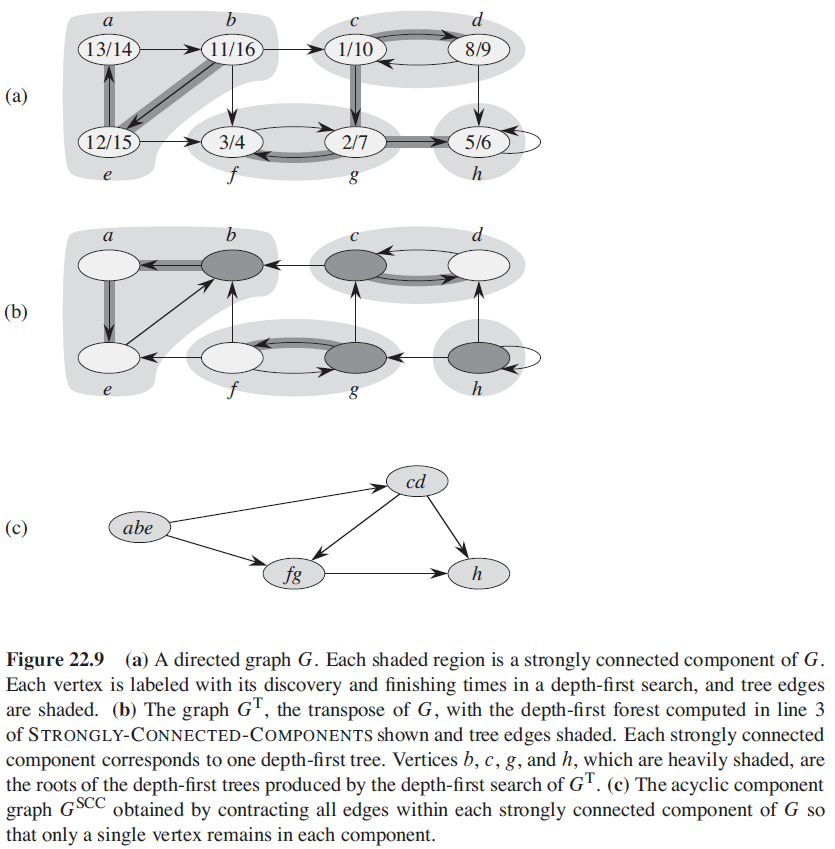## 最小生成树

 1 2 3 4 5 6 7 8  // 在每遍循环之前，A 是某棵最小生成树的一个子集 // 加入集合 A 而不会破坏 A 的循环不变式的边称为集合 A 的安全边 GENERIC-MST(G, w) A = ∅ while A does not form a spanning tree find an edge(u, v) that is safe for A A = A ∪ {(u, v)} return A 

### Kruskal 算法

  1 2 3 4 5 6 7 8 9 10  MST-KRUSKAL(G, w) A = ∅ for each vertex v in G.V MAKE-SET(v) sort the edge of G.E into nondecreasing order by weight w for each edge (u, v) in G.E, taken in nondecreasing order by weight if FIND-SET(u) ≠ FIND-SET(v) A = A ∪ {(u, v)} UNION(u, v) return A 

Kruskal 算法的时间复杂度为 $O(ElgV)$。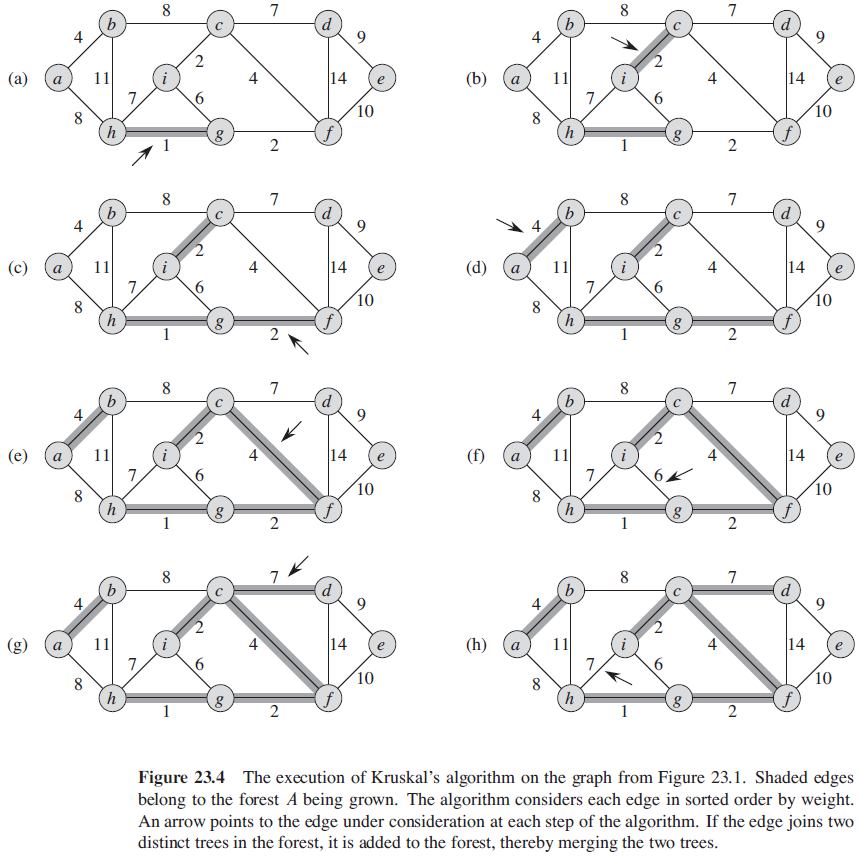### Prim 算法

  1 2 3 4 5 6 7 8 9 10 11 12  MST-PRIM(G, w, r) for each u in G.V u.key = ∞ u.π = NIL r.key = 0 Q = G.V while Q ≠ ∅ u = EXTRACT-MIN(Q) for each v in G.Adj[u] if v in Q and w(u, v) < v.key v.π = u v.key = w(u, v)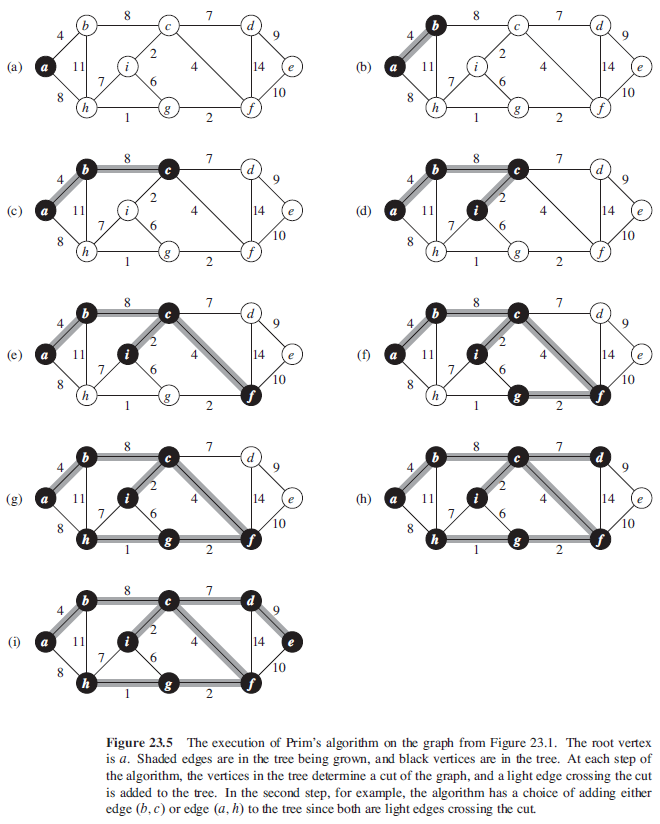## 单源最短路径

1. 最短路径的子路径也是最短路径
2. 如果从结点 s 到结点 v 的某条路径上存在权重为负值的环路，我们定义 $\delta(s, v) = -\infty$
3. 一般地，我们假定在找到的最短路径中没有环路，即它们都是简单路径。
  1 2 3 4 5 6 7 8 9 10 11 12 13 14  /** 初始化 * v.d：s 到 v 的最短路径估计 * v.π：前驱结点 */ INITIALIZE-SINGLE-SOURCE(G, s) for each vertex v in G.V v.d = ∞ v.π = NIL s.d = 0 // 松弛操作 RELAX(u, v, w) if v.d > u.d + w(u, v) v.d = u.d + w(u, v) v.π = u 

Dijkstra 算法和用于有向无环图的最短路径算法对每条边仅松弛一次。 Bellman-Ford 算法则对每条边松弛 |V| - 1 次。

### Bellman-Ford 算法

Bellman-Ford 算法解决的是一般情况下的最短路径问题。该算法返回 TRUE 值当且仅当输入图不包含可以从源结点到达的权重为负值的环路。

 1 2 3 4 5 6 7 8 9  BELLMAN-FORD(G, w, s) INITIALIZE-SINGLE-SOURCE(G, s) for i = 1 to |G.V|-1 for each edge (u, v) in G.E RELAX(u, v, w) for each edge (u, v) in G.E if v.d > u.d + w(u, v) return FALSE return TRUE 

Bellman-Ford 算法的总运行时间为 O(VE)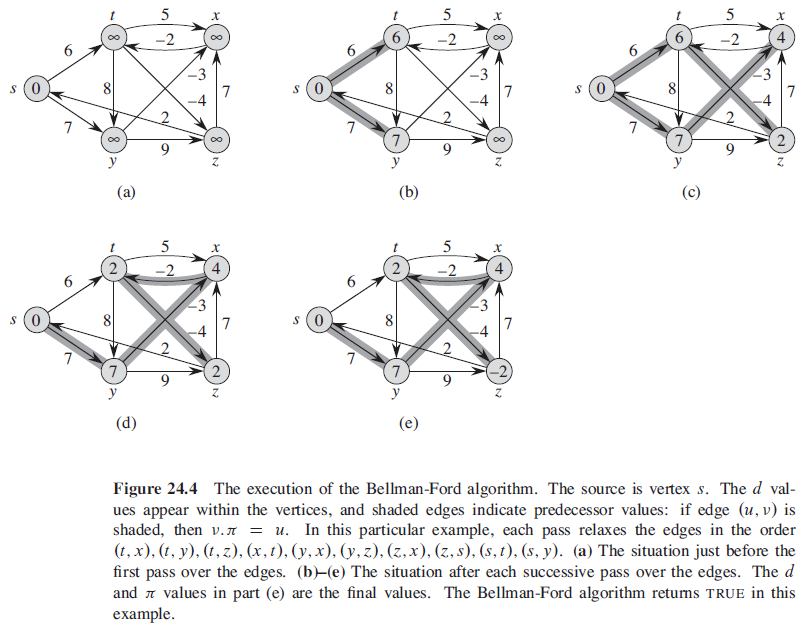### 有向无环图中的单源最短路径问题

 1 2 3 4 5 6  DAG-SHOPTEST-PATHS(G, w, s) topologically sort the vertice of G INITIALIZE-SINGLE-SOURCE(G, s) for each vertex u, taken in topologically sorted order for each vertex v in G.Adj[u] RELAX(u, v, w)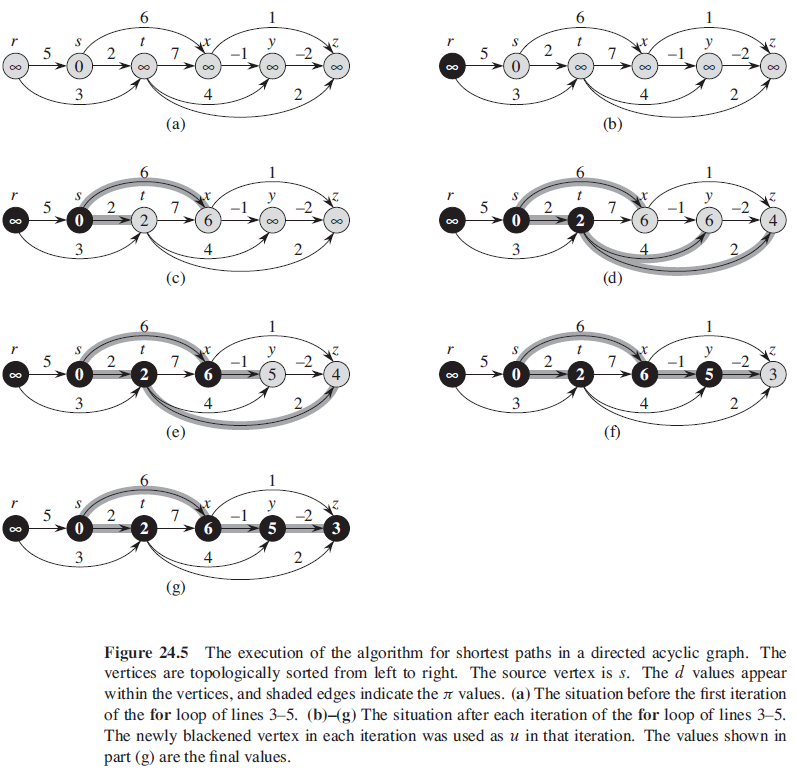## Dijkstra 算法

Dijkstra 算法解决的是带权重的有向图上的单源最短路径问题，该算法要求所有边的权重都为非负值。

 1 2 3 4 5 6 7 8 9  DIJKSTRA(G, w, s) INITIALIZE-SINGLE-SOURCE(G, s) S = ∅ Q = G.V while Q ≠ ∅ u = EXTRACT-MIN(Q) S = S ∪ {u} for each vertex v in G.Adj[u] RELAX(u, v, w)